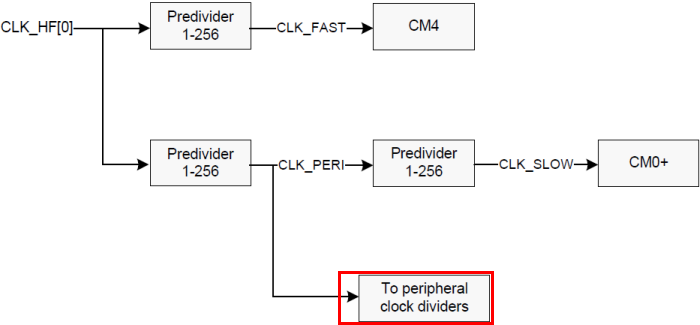PSoC 6 Peripheral Driver Library
Peripherals Clock Dividers

## General Description

There are multiple peripheral clock dividers that, in effect, create multiple separate peripheral clocks.

The available dividers vary per device series. As an example, for the PSoC 63 series there are 29 dividers:

• eight 8-bit dividers
• sixteen 16-bit dividers
• four fractional 16.5-bit dividers (16 integer bits, 5 fractional bits)
• one fractional 24.5-bit divider (24 integer bits, 5 fractional bits)

The 8-bit and 16-bit dividers are integer dividers. A divider value of 1 means the output frequency matches the input frequency (that is, there is no change). Otherwise the frequency is divided by the value of the divider. For example, if the input frequency is 50 MHz, and the divider is value 10, the output frequency is 5 MHz.

The five fractional bits supports further precision in 1/32nd increments. For example, a divider with an integer value of 3 and a fractional value of 4 (4/32) results in a divider of 3.125. Fractional dividers are useful when a high-precision clock is required, for example, for a UART/SPI serial interface.Each peripheral can connect to any one of the programmable dividers. A particular peripheral clock divider can drive multiple peripherals.

The SysClk driver also supports phase aligning two peripheral clock dividers using Cy_SysClk_PeriphEnablePhaseAlignDivider(). Alignment works for both integer and fractional dividers. The divider to which a second divider is aligned must already be enabled.

## API Reference

Functions

Enumerated Types